## RD Sharma Class 8 Solutions Chapter 7 Factorizations Ex 7.6

These Solutions are part of RD Sharma Class 8 Solutions. Here we have given RD Sharma Class 8 Solutions Chapter 7 Factorizations Ex 7.6

Other Exercises

Factorize each of the following algebraic expressions :
Question 1.
4x2 + 12xy + 9y2
Solution:
4x2 + 12xy + 9y2 = (2x)2 + 2 x 2x x 3y + (3y)2 {∵ a2 + 2ab + b2 = (a +b)2}
= (2x + 3y)2

Question 2.
9a2 – 24ab + 16b2
Solution:
9a2 – 24ab + 16b2
= (3a)2 – 2 x 3a x 4b + (4b)2     {∵ a2 – 2ab + b2 = (a – b)2}
= (3a – 4b)2

Question 3.
36a2 – 6pqr + 9r2
Solution:
p2q2 – 6pqr + 9r2
= (pq)2 – 2 x pq x3r + (3r){∵ a2 – 2ab + b2 = (a -b)2}
= (pq-3r)2

Question 4.
36a2 + 36a + 9
Solution:
36a2 + 36a + 9
= (6a)2 + 2 x 6a x 3 + (3)2   {∵ a2 + 2ab + b2 = (a + b)2
= (6a + 3)2

Question 5.
a2 + 2ab + b2 – 16
Solution:
a2 + 2ab + b2 – 16
= (a + b)2 – (4)2     {∵ a2 + 2ab + b2 = (a + b)2 and a2 – b2 = (a + b) (a – b)}
= (a + b + 4) (a + b – 4)

Question 6.
9z2 – x2 + 4xy – 4y2
Solution:
9z2 – x2 + 4xy – 4y2    {∵ a2 – b2 = (a + b) (a – b) and a2 – 2ab + b2 (a – b)2}
= 9z2 – (x2 – 4xy + 4y2)
= (3z)2 – [(x)2 – 2 x x x 2y + (2y)2]
= (3z)2-(x-2y)2
= (3z + x – 2y) (3z – x + 2y)

Question 7.
9a4 – 24a2b2 + 16b4 – 256
Solution: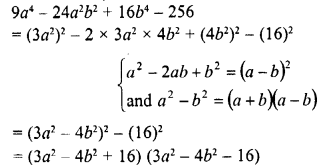Question 8.
16 – a6 + 4a3b3 – 4b6
Solution: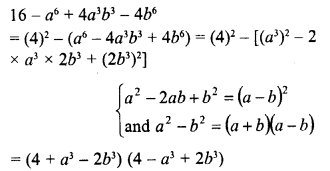Question 9.
a2 – 2ab + b2 – c2
Solution: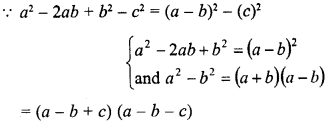Question 10.
x2 + 2x + 1 – 9y2
Solution:Question 11.
a2 + 4ab + 3b2
Solution:
a2 + 4ab + 3b2
= a2 + 4ab+ 4b2 – b2
= (a)2 + 2 x a x 2b + (2b)2 – b(∵ 3b2 = 4b2 – b2)
= (a + 2b)2 – (b)2   {∵ a2 – b2 = (a +b) (a – b)}
= (a + 2b + b) (a + 2b- b)

Question 12.
96 – 4x-x2
Solution:
96 – 4x – x2 = 96 – (4x + x2)
= 96 – [(x)2 + 2 x x x 2 + (2)2] + (2)2   (on completing the square)
= 96 + 4 – (x + 2)2 = 100 – (x + 2)2
= (10)2 – (x + 2)2
= (10 + x + 2) (10 – x- 2)
= (x + 12) (-x + 8)

Question 13.
a4 + 3a2 + 4
Solution:
a4 + 3a2 + 4
= (a2)2 + (2)2 + 2 x a2 x 2 – a2   (on completing the square)
= (a2 + 2)2 – (a)2
= (a2 + 2 + a) (a2 + 2 – a)
= (a1 + a + 2) (a2 – a + 2)

Question 14.
4a4 + 1
Solution:
4x4 + 1 = (2a2)2 + (1)2 + 2 x 2x2 x 1 – 2 x 2xx 1  (completing the square)
= (2x2 + 1)2 – 4a2
= (2x2 + 1)2 – (2a)2   {a2 – b2 = (a + b) (a – b)}
= (2x2 + 1 + 2a) (2a2 + 1 – 2a)
= (2a2 + 2a + 1) (2a2 – 2a + 1)

Question 15.
4x4+y4
Solution:
4a4 + y4 = (2x2)2 + (y2)2 + 2 x 2x2y2 – 2 x 2x2y2
= (2x2 + y2)2 – 4x2y2
= (2x2 + y2)2 – (2xy)2
= (2x2 + y2 + 2xy) (2x2 + y2 – 2xy)
= (2x2 + 2xy + y2) (2x2 – 2xy + y2)

Question 16.
(x+ 2)2 – 6 (a + 2) + 9
Solution:
(x + 2)2 – 6 (x + 2) + 9
=  (x + 2)2 – 2 x (x + 2) x 3 + (3)2
= (x + 2 – 3)2
= (x-1)2 = (x-1)(x-1)

Question 17.
25 – p2 – q2 – 2pq
Solution: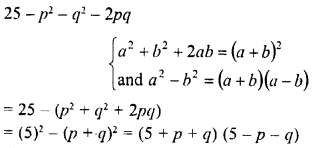Question 18.
a2 + 9y2 – 6xy – 25a2
Solution:
a2 + 9y2 – 6xy – 25a2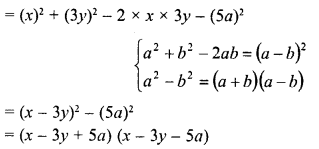Question 19.
49 – a2 + 8ab – 16b2
Solution:Question 20.
a2 – 8ab + 16b2 – 25c2
Solution: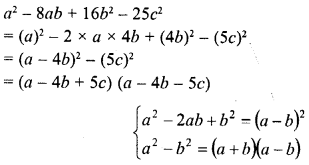Question 21.
x2 -y2+ 6y- 9
Solution: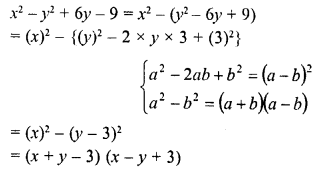Question 22.
25x2 – 10x + 1 – 36y2
Solution: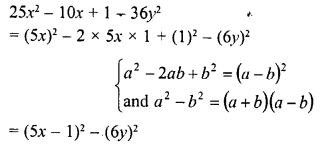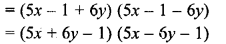Question 23.
a2-b2 + 2bc – c2
Solution: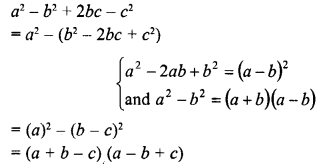Question 24.
a2 + 2ab + b2 -c2
Solution: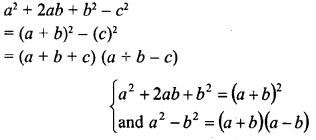Question 25.
49 -x2 – y2 + 2xy
Solution: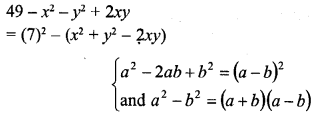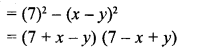Question 26.
a2 + 4b24ab 4c2
Solution: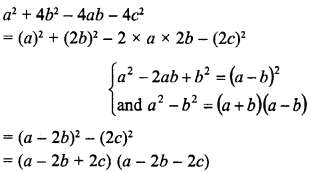Question 27.
x2 -y24xz + 4z2
Solution: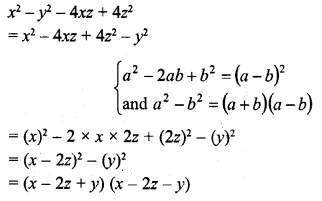Hope given RD Sharma Class 8 Solutions Chapter 7 Factorizations Ex 7.6 are helpful to complete your math homework.

If you have any doubts, please comment below. Learn Insta try to provide online math tutoring for you.